# GMAT Quant: Geometry – Volume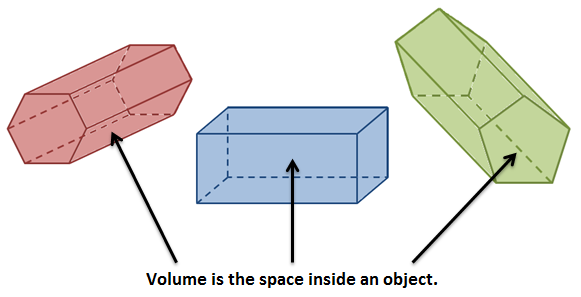Quant in GMAT exam is the most scoring and No, it is not time consuming if you have a good hold of it. The mantra is to know all basics and formulas and practice a lot.

## What is Meant by Volume?

In a 3-D shape, amount of space occupied by an object, is known as volume. The unit of volume is cubic units, liters etc.

Let’s check some standard volume formula for some regular shapes:

1. Cube

Cube is a special form of rectangular solid whose all six sides are equal.

Volume = a

Where, a = length of the sides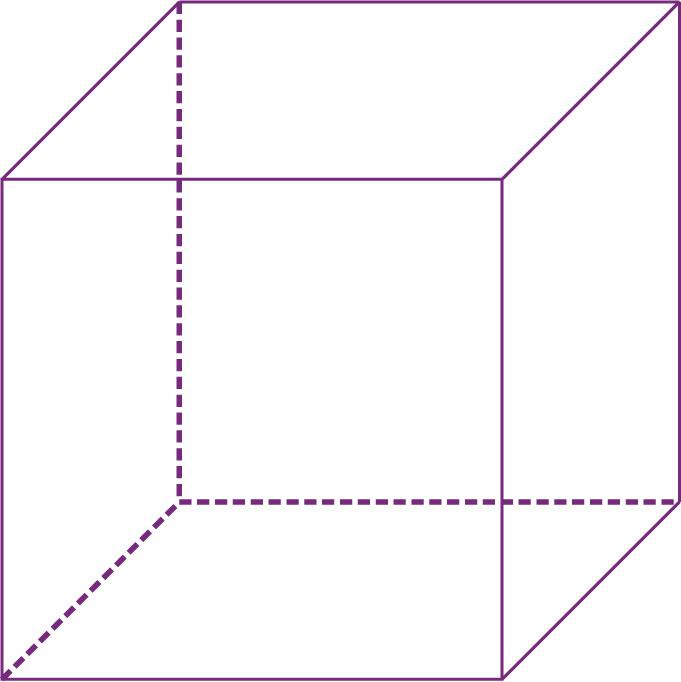2. Volume of Rectangular Solids

All the sides of a rectangular solid are rectangular in shape. If all the six sides have the same length then it is known as a cube otherwise, it is a cuboid.Volume of rectangular solid = lwh =length ×weidth ×height

3. Volume of Cylinder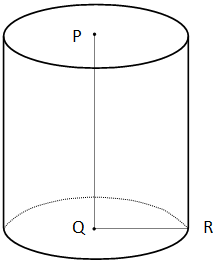Volume, $V = \pi r^{2} h = \pi \times radius \times radius \times height$

4. Volume of Sphere

Sphere is a 3- dimensional form of a circle

Volume, $V = \frac{4}{3}\pi r^{3}$5.Right Circular Cone, $V = \frac{1}{3}\pi r^{2}h$6.Ellipsoid is the 3 –D formation of an ellipse.

Volume, $V = \frac{4}{3} \pi \times radius_1 \times radius_2 \times radius_3$

Volume, $V = \frac{1}{3} \times base \times height$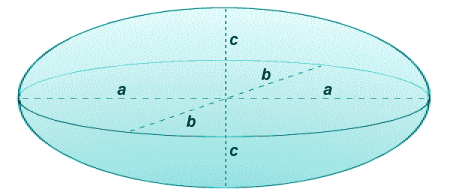7.Tetrahedron: A tetrahedron is a polyhedron and type of pyramid which has a triangular base. It has four triangular faces, four vertex corners, and six straight edges.

Volume, $V = \frac{a^{3}}{6\sqrt{2}}$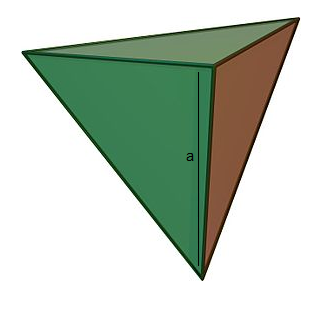Let’s solve a problem and be prepared to tackle any problem offered by GMAT.

Question:

The inside dimensions of a rectangular wooden box are 6 inches by 8 inches by 10 inches. A cylindrical canister is to be placed inside the box so that it stands upright when the closed box rests on one of its six faces. Of all such canisters that could be used, what is the radius, in inches, of the one that has maximum volume?

1. 3
2. 4
3. 5
4. 6
5. 8

Solution:

Cylinder can be placed in a rectangular box on three different bases.

Base1. Height = 10

r = 3

$V = \pi r^{2}h$ $V = \pi \times 3^{2} \times 10$ $V = 90 \pi$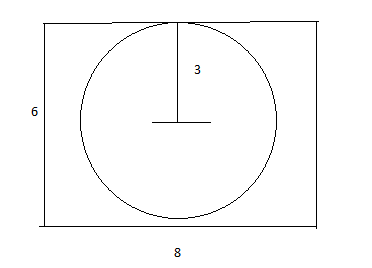Base 2: height = 6

r = 4

$V = \pi r^{2}h$ $V = \pi \times 4^{2} \times 6$ $V = 96 \pi$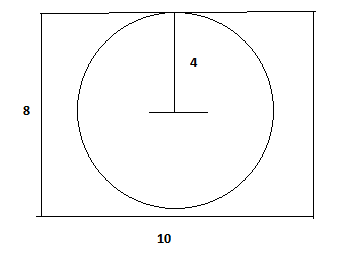Base = 3; Height = 8

r = 3

$V = \pi r^{2}h$ $V = \pi \times 3^{2} \times 8$ $V = 72 \pi$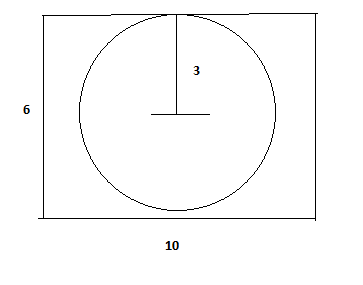Question: There is a shower from which 10 cm of rain falls. Find the volume of water that will fall on the ground in an area of 3.5 hectares.

1. 35 m3
2. 350 m3
3. 3500 m3
4. 35000 m3

Solution: 1 hectare = 10, 000 m2

So total area $= 3.5 \; hectares = 3.5 \times 10,000 = 35,000 m^{2}$

According to question, $Depth = \frac{10}{100}m = 0.1 m$

So, volume, $V = Depth \times Area = 35000 \times 0.1 = 3500m^{3}$